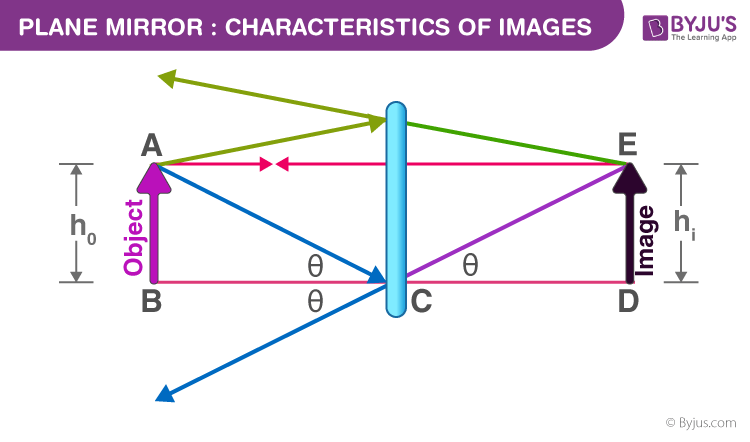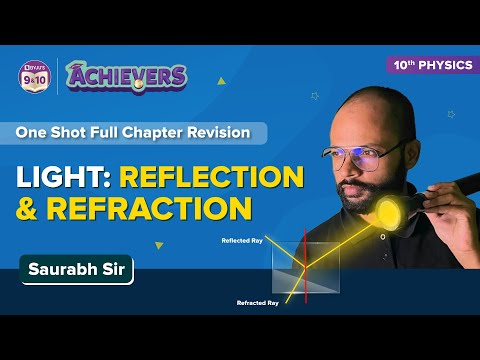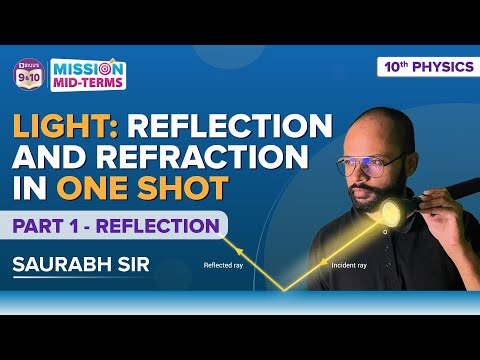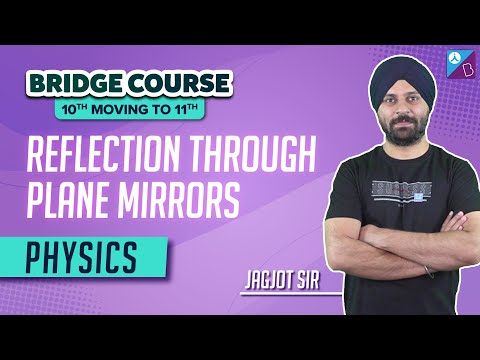# Plane Mirror : Characteristics of Images - Mirrors

When a ray of light falls on a surface, then it can undergo one of the following three phenomena reflection, refraction, or absorption. When it falls on a normal surface then most of the light gets absorbed. So mirrors are polished surfaces coated with mercury such that they reflect most of the light falling on them. Now based on the type of reflecting surface we can classify mirrors as concave, convex, or plane mirrors. Here we will be talking about the plane mirror only. So to form an image we require at least two rays from the object which meet or appear to meet at a point. In the case of a plane mirror, here we have used three rays for better clarity in the ray diagram shown below.

## Formation of Image by a Plane MirrorFor the ray starting from point A and travelling in a horizontal direction towards point E, the angle of incidence is 0, and hence it retraces its path. Similarly, the ray starting from A and travelling towards point C follows the law of reflection. When these rays are produced backwards, they appear to meet at a point E. Now this image is known as a virtual image. What is the difference between real and virtual images? In a real image, the rays of light actually meet after reflection, while in a virtual image, it appears to meet but do not actually meet. A real image can be obtained on screen but not a virtual image.Following is the table explaining other concepts related to the mirror:

### Characteristics of the image formed by a plane mirror :

From the above diagram, the following characteristics of the image can be observed.

• It is virtual
• It is erect and of the same size as the object
• The distance of the object from the plane mirror is the same as the distance of the image from the plane mirror.
• It is laterally inverted.

One of the important characteristics of the image is that it is laterally inverted. It means if you raise your left hand it would appear in the plane mirror that you have raised your right hand.

## Watch this video to understand the significance of the mirror formula, which is also applicable to the plane mirror### Laws of Reflection

There are two laws of reflection which are always followed by plane mirror and they are:

• The angle of incidence and the angle of reflection are always equal.
• The incident ray, the normal at the point, and the reflected ray, all lie on the same plane.

### Properties of an Image Formed by Plane Mirror

• The image obtained is virtual.
• The image is laterally inverted.
• The image is erect.
• The size of the image is the same as the size of the object.
• The distance between the image obtained from the mirror is the same as the distance between the object from the mirror.

#### Watch the video and revise all the important concepts in the chapter Light Reflection and Refraction Class 10#### The below video helps to revise the topic reflection in the chapter Light Reflection and Refraction Class 10## Frequently Asked Questions – FAQs

Q1

### What is the magnification of the images formed by the plane mirror?

As the size of the object and image are the same, the magnification (ratio of image size to the object size) is equal to +1.
Q2

### Can a plane mirror form a real image?

A plane mirror only forms virtual images of real objects. However, a plane mirror can form a real image of a virtual object when hit by a converging beam of light.
Q3

### If a ray falls normally on a plane mirror, what is the angle of incidence?

The angle of incidence will be 0°.
Q4

### What is the focal length of a plane mirror?

The focal length of a plane mirror is infinite.
Q5

### If a man stands 1m away from the plane mirror, what is the distance of the man from his image?

For the plane mirror, the image distance is always equal to the object distance. So, the total distance between the man and his image will be (1+1) = 2m.

## Watch the video below to learn about the reflection on a plane mirrorStay tuned with BYJU’S to learn about the application of the different types of mirrors, spherical mirrors, lenses, etc.

Test your knowledge on Plane Mirrors